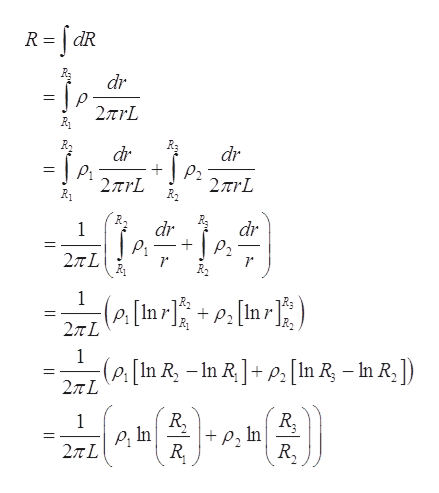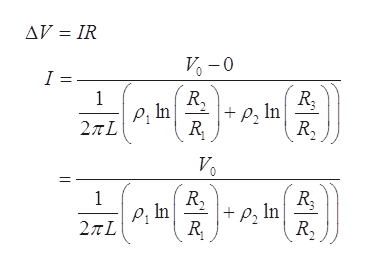# hollow section0 to RR

Question

1. A hollow cylinder of length L, inner radius R1 , and radius Ris filled with two different materials. From Rto R2, the space is filled with a material of resistivity p. From Rto R, the space is filled with material of resistivity p

a) Derive an equation for the resistance of this structure as current flows from Rto R3 (ALONG the radial direction, NOT along the length). Ignore edge effects.

b) If the total voltage across the object is Vo, find an expression for the current density along the objects ( i.e. find J(r)).

Take V= Vo  at R1   and  V=0 at R3

check_circleExpert Solution
Step 1

(a)

Formula for the resistance is:

Step 2

Consider an elemental strip of thickness dr and density rho. Here the area will be the area of the surface of the thin cylinder that is 2pirl.

Therefore, from definition of resistance. The expression of the resistance of the elemental strip is:help_outlineImage TranscriptioncloseR dR dr 2TrL dr dr 2TrL R2 2rL dr dr 2TL 1 (Ps[In r] P2 27TL 1 -(P[In R, -In RP[In R - In R, ]) 27TL R In R. 1 In R, fullscreen
Step 3

(b)

From ohm&rsqu...help_outlineImage TranscriptioncloseAV IR V-0 I = 1 p, In R P, In 2πL R 1 PIn 2TL fullscreen

### Want to see the full answer?

See Solution

#### Want to see this answer and more?

Solutions are written by subject experts who are available 24/7. Questions are typically answered within 1 hour*

See Solution
*Response times may vary by subject and question
Tagged in

### Physics